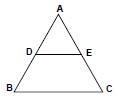# Classification of Triangles | Properties of a Triangle for IBPS PO exam preparation## Triangle : Classification of Triangles | Properties of a Triangle for IBPS PO exam preparation

Classification of Triangles:

On the basis of length of the sides, triangles can be classified into three categories:

• Scalene: Having all the length of its sides unequal.
• Isosceles: Having any two sides of the same length.
• Equilateral: Having all the three sides of the triangle of equal length.

On the basis of angles, triangles can be classified into three categories:

• Obtuse-angled triangle: Largest angle of the triangle is greater than 90 degree.
• Acute angled triangle: All angles of the triangle is less than 90 degree.
• Right-angled Triangle: Largest angle of the triangle is equal to 90 degree.

Properties of a Triangle:

1. Sum of all the three angles of the triangle is equal to 180 degree.

2. An exterior angle of the triangle is equal to the sum of the interior opposite angles.

3. The sum of any two sides of the triangle is always greater than the third side.

4. The difference between any two sides of the triangle is always less than the third side.

5. The side opposite to the greatest angle is the greatest side and the side opposite to the smallest angle is the shortest side.

Practice with mock test papers for Bank PO & Clerk, click on online classes for banking.

Mid-Point Theorem:

A line joining the midpoints of any two sides of a triangle is parallel and equal to half of the third side.If in Δ ABC, D & E are the midpoints of AB & AC respectively, then we have

DE || BC and DE = ½ BC

### Similar triangles:

Two triangles are said to be similar if they have the same shape but not the same size.

NOTE: Congruent triangles are always similar but similar triangles need not be congruent.

Online test series for IBPS PO & Clerk is available, click on online banking coaching classes for details.

Properties of similar triangles

If two triangles are similar, the following properties hold true.

(a) The ratio of the medians is equal to the ratio of the corresponding sides.

(b) The ratio of the altitudes is equal to the ratio of the corresponding sides.

(c) The ratio of the internal bisectors is equal to the ratio of corresponding sides.

(d) The ratio of inradii is equal to the ratio of the corresponding sides.

(e) The ratio of the circumradii is equal to the ratio of the corresponding sides.

(f) The ratio of the area is equal to the ratio of squares of the corresponding sides.

(g) The ratio of the area is equal to the ratio of squares of the corresponding medians.

(h) The ratio of the area is equal to the ratio of the squares of the corresponding altitudes.

(i) The ratio of the area is equal to the ratio of the squares of the corresponding angle bisectors.

Basic Proportionality Theorem

In a triangle, if a line drawn parallel to one side of a triangle divides the other two sides in the same ratio.So, if DE is drawn parallel to BC, it would divide sides AB and AC proportionally i.e.

Pythagoras Theorem:

The square of the hypotenuse of a right-angled triangle is equal to the sum of the squares of the other two sides i.e. in a right-angled triangle ABC, right angled at B,

AC2 = AB2 + BC2

For more articles, video lectures, banking exams pattern, online test series, register with Takshila Learning.

Register yourself with Takshila Learning for more articles on other subjects, Bank PO & Clerk online digital classes, online test series for bank PO, & much more.

Takshila Learning is an e-learning solution for banking aspirants where you can go through our online study material & video lectures from anywhere and at any time. You can also give online mock tests of SBI, IBPS PO, Clerk, RRB, etc.

Online classes for banking include:

• Complete coverage of the whole syllabus
• Interactive video sessions by our experienced faculty
• Doubt sessions
• Chapter wise tests conducted regularly
• Online Test Series with complete analysis
• Current affairs

Follow us on a Social media

## Call us: 8800999280/8800999284 orfill the form for any other details:

### Share and Enjoy !

0Shares
November 23, 2018

### 0 responses on "Classification of Triangles | Properties of a Triangle for IBPS PO exam preparation"